#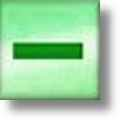Subtraction Worksheets

## Dynamically Created Subtraction Worksheets

Here is a graphic preview for all of the subtraction worksheets. You can select different variables to customize these subtraction worksheets for your needs. The subtraction worksheets are randomly created and will never repeat so you have an endless supply of quality subtraction worksheets to use in the classroom or at home. Our subtraction worksheets are free to download, easy to use, and very flexible.

## Quick Link for All Subtraction Worksheets

Click the image to be taken to that Subtraction Worksheet.

##### Subtraction Facts TablesSubtraction Worksheets##### Single DigitSubtraction Worksheets##### Zero to TwentySubtraction Worksheets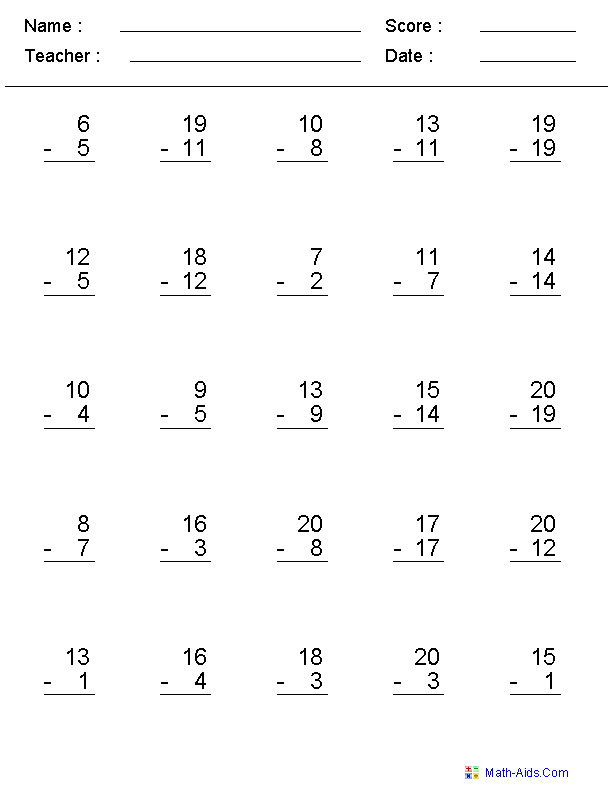##### Zero to 99Subtraction Worksheets##### Subtracting Within a NumberSubtraction Worksheets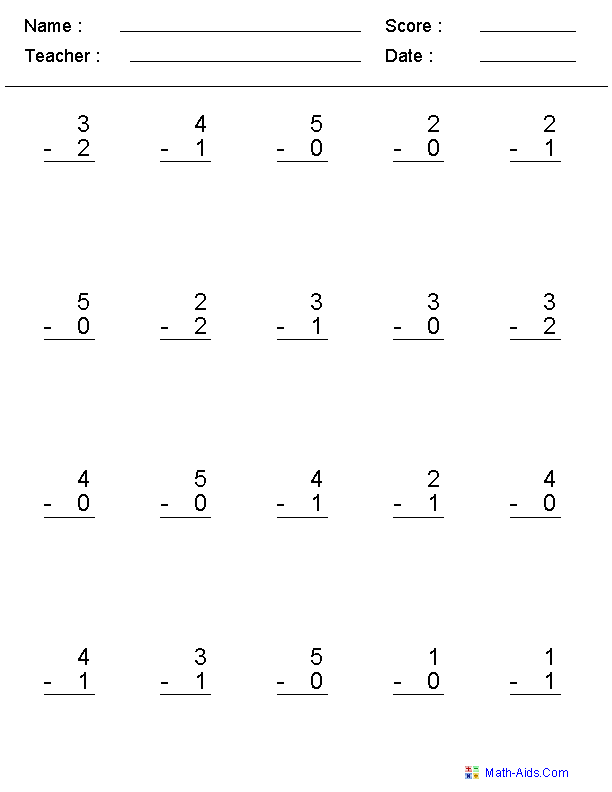##### Subtracting with DotsSubtraction Worksheets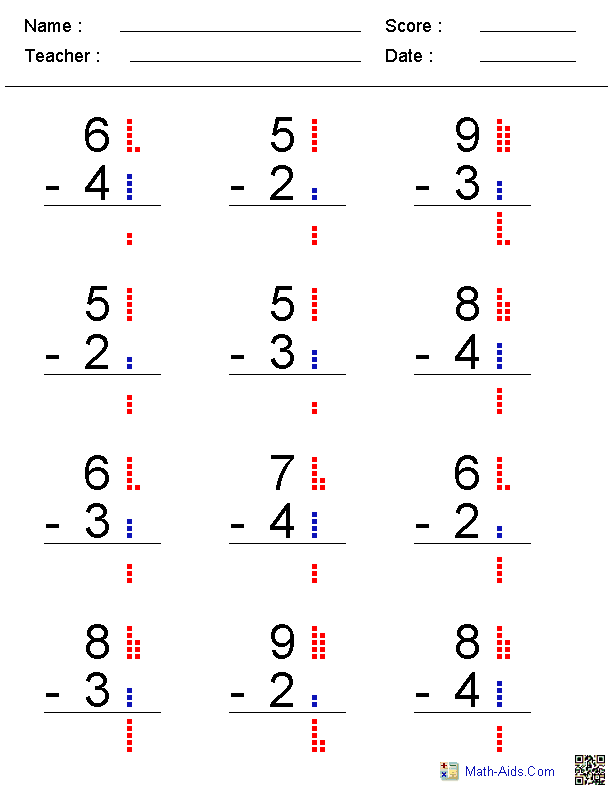##### Subtracting Dot Figures to TenSubtraction Worksheets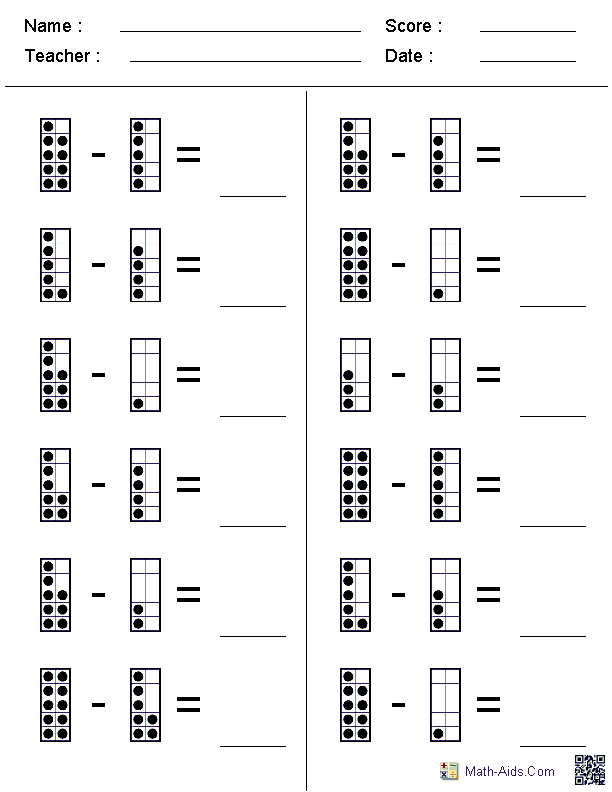##### Subtracting Dot Figures to 20Subtraction Worksheets##### Subtracting Two Digit NumbersEnding in a Fixed Number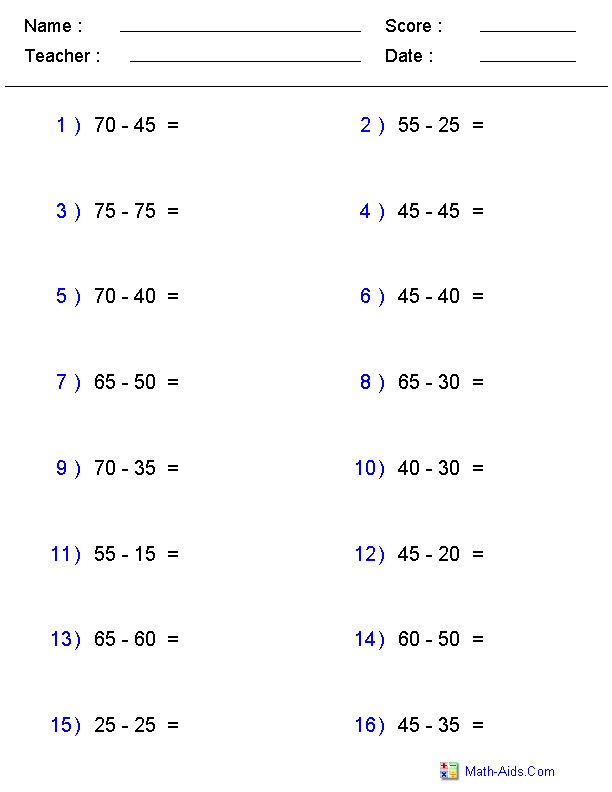##### Single or Multi DigitSubtraction Worksheets##### 2, 3, or 4 DigitsSubtraction Worksheets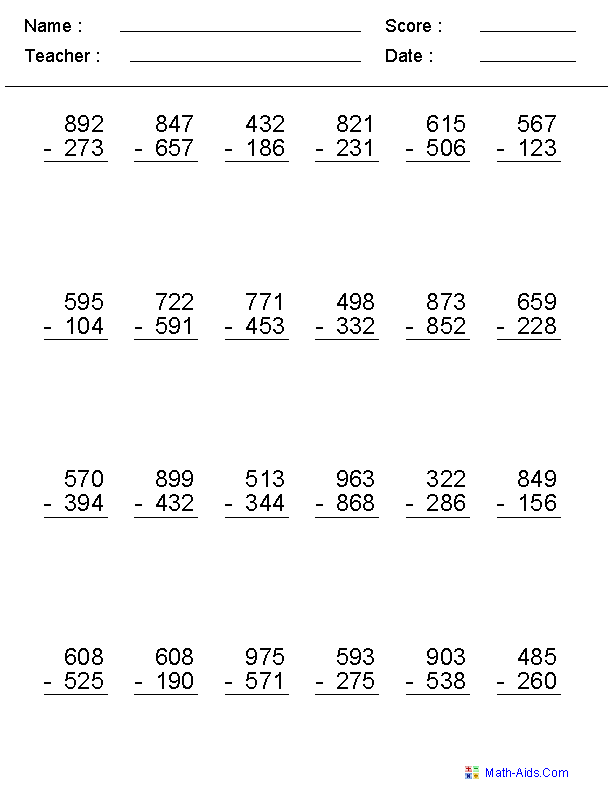##### 4, 5, or 6 DigitsSubtraction Worksheets##### 2 Digit No RegroupSubtraction Worksheets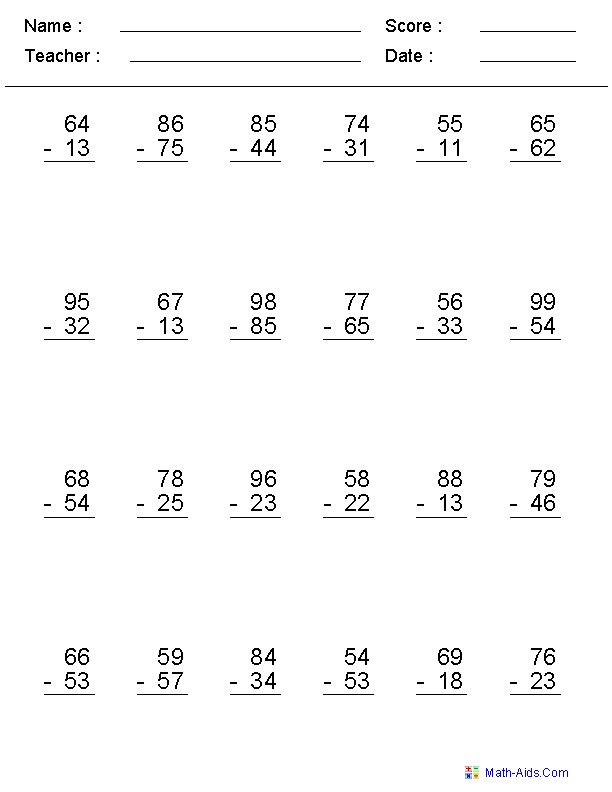##### 2, 3, or 4 Digit No RegroupSubtraction Worksheets##### No Regroup - Horizontal FormatSubtraction Worksheets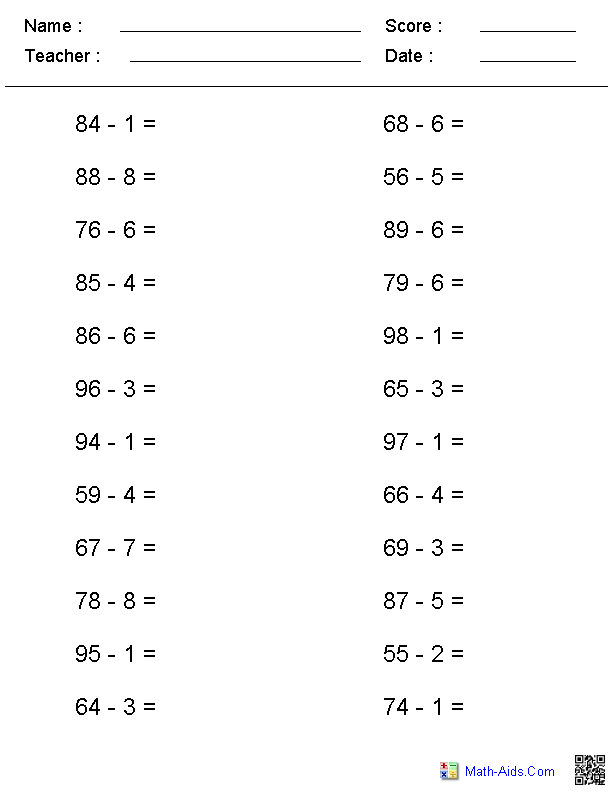##### Subtraction Across ZeroSubtraction Worksheets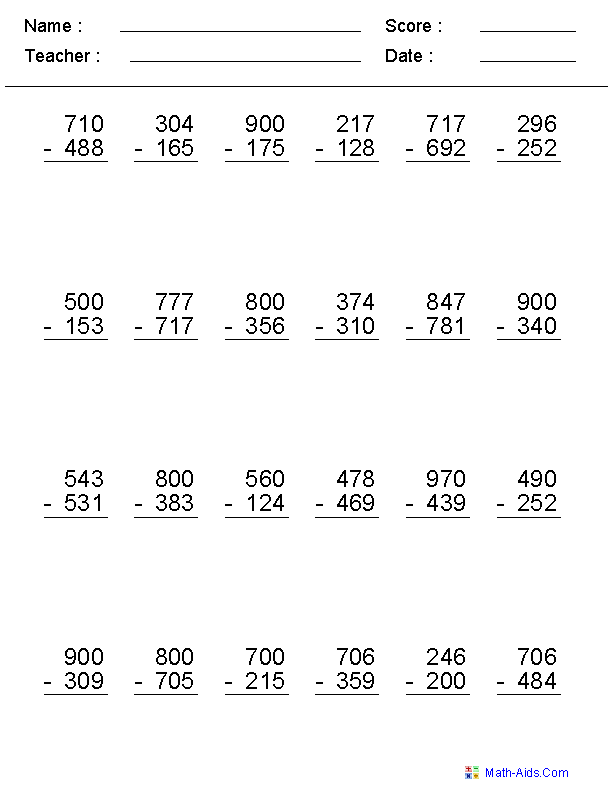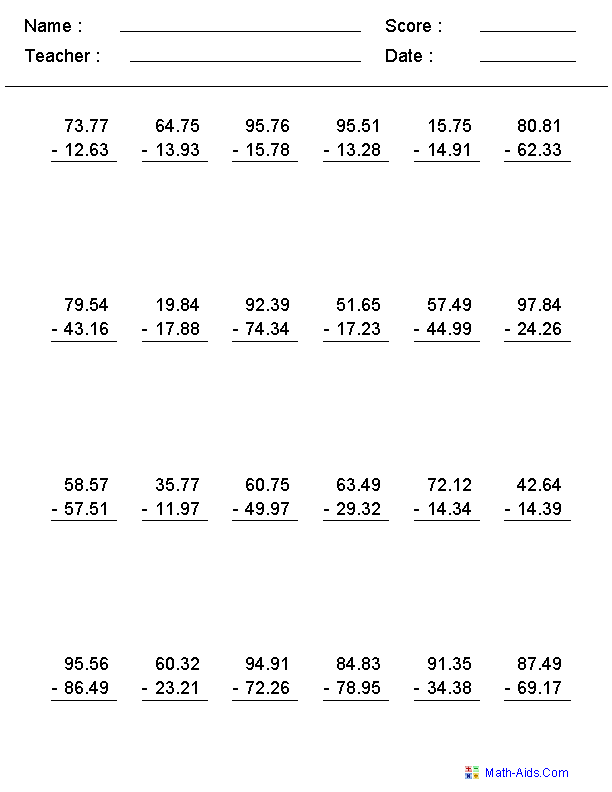##### Subtracting MoneySubtraction Worksheets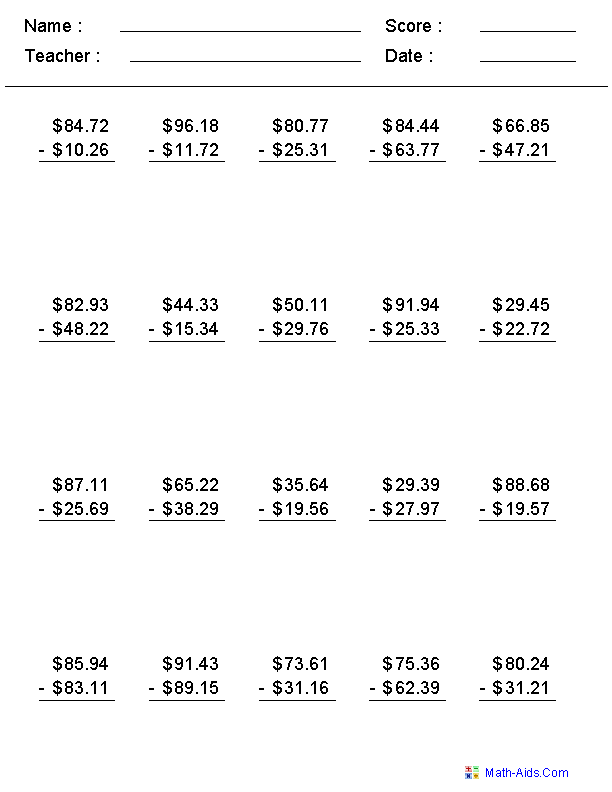##### 1, 3, or 5 Minute DrillsSubtraction Worksheets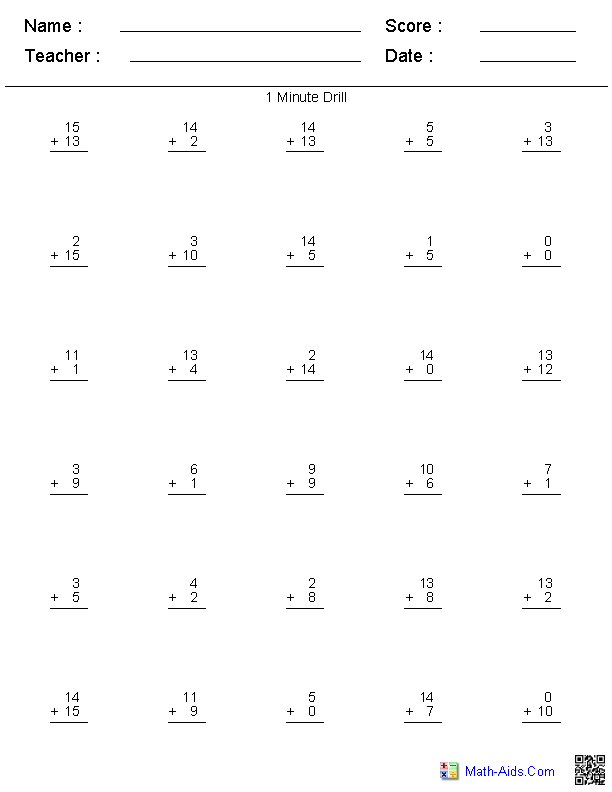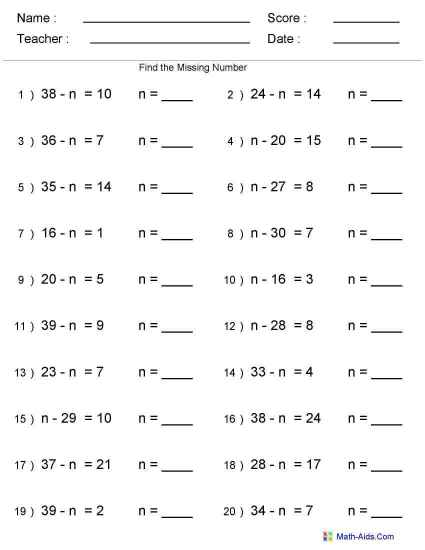##### Missing DigitsSubtraction Worksheets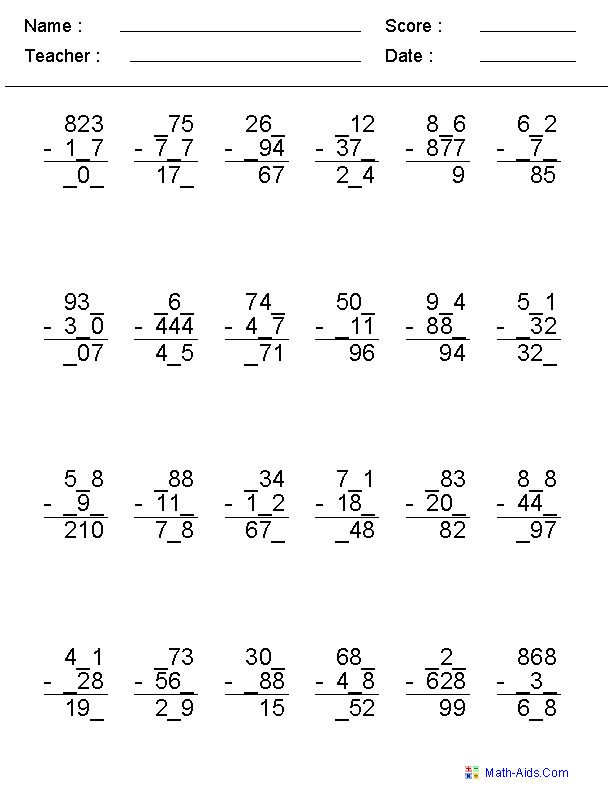##### Irregular UnitsSubtraction Worksheets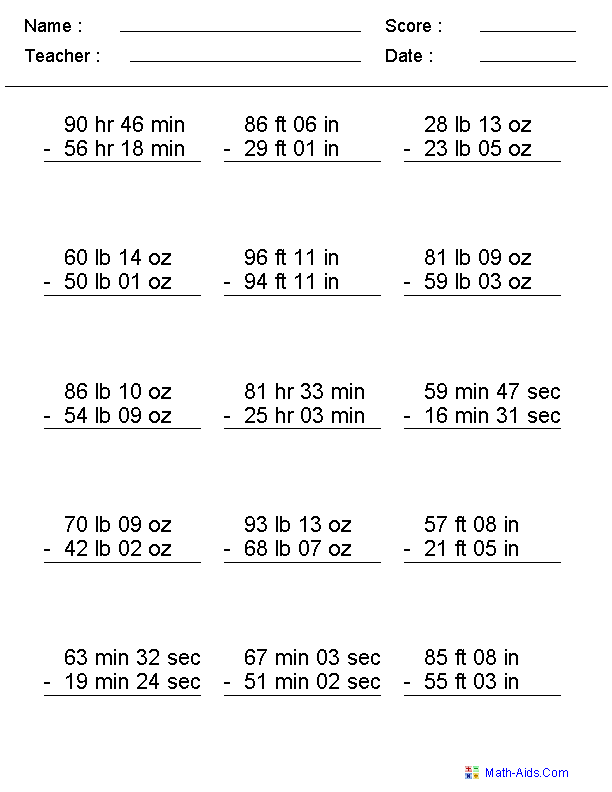##### Subtracting Feet & InchesSubtraction Worksheets##### Subtracting DoublesSubtraction Worksheets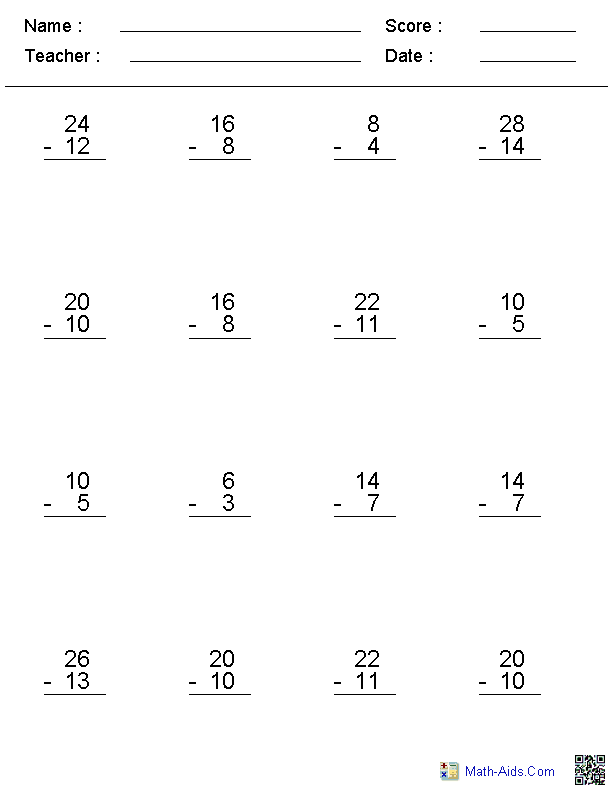##### Subtraction FactsOne to Ten Worksheets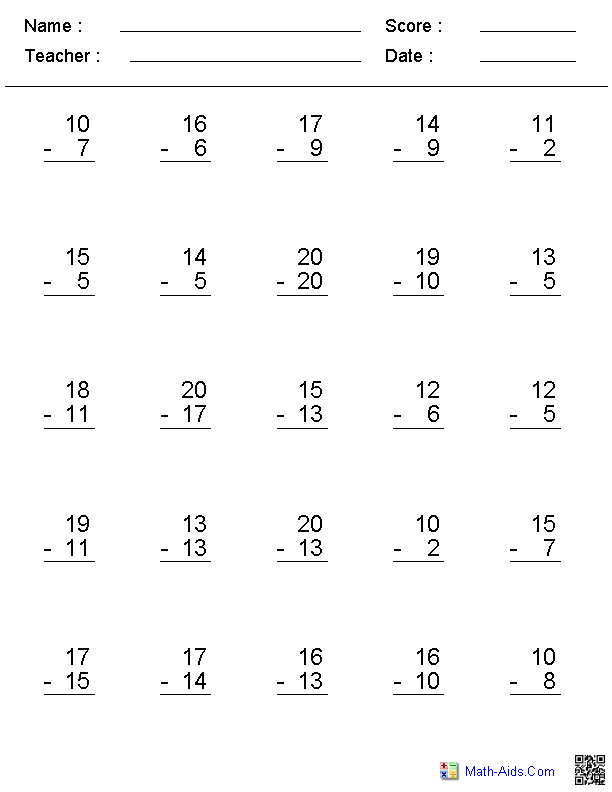##### Visual SubtractionSubtraction Worksheets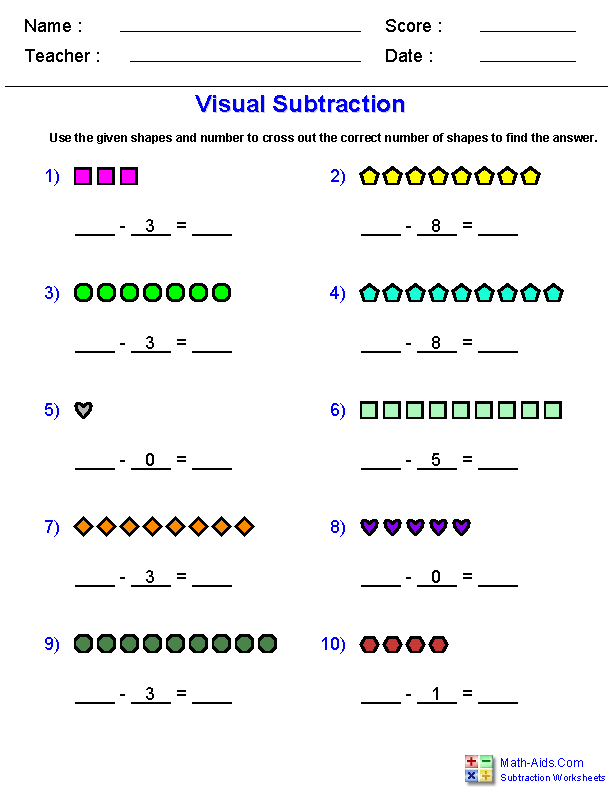Recommended Videos

## Detailed Description for All Subtraction Worksheets

Subtraction Facts Table Worksheets
These subtraction facts table are colorful and a great resource for teaching kids their subtraction facts. A complete set of printable subtraction facts for 1 to 12.

Single Digit Subtraction Worksheets
Vertical Format | 2 Numbers

These single digit subtraction worksheets are configured for 2 numbers in a vertical problem format. The range of numbers used for each worksheet may be individually varied to generate different sets of subtraction worksheets. You may select up to 30 problems for these worksheets.

Zero to Twenty Subtraction Worksheets
Vertical Format | 2 Numbers

These subtraction worksheets are configured for 2 numbers in a vertical problem format where you may select numbers from zero to twenty to generate different sets of subtraction problems. You may select up to 30 problems for these worksheets.

Zero to 99 Subtraction Worksheets
Vertical Format | 2 Numbers

These subtraction worksheets are configured for 2 numbers in a vertical problem format where you may select numbers from zero to 99 to generate different sets of subtraction problems. You may select up to 30 problems for these worksheets.

Subtracting Within a Number Worksheets
Vertical Format | 2 Numbers

These subtraction worksheets will produce vertical format subtraction problems within a selected number. You may select the maximum number and the number of problems to produce, or you may select the non repeating Kindergarten, 1st, or 2nd Grade set of unique problems. These special problem set will produce worksheets that will directly test the Common Core State Standards.

Subtracting with Dots Subtraction Worksheets
Vertical Format | 2 Numbers

These subtraction worksheets will produce 12 vertical subtraction problems with dots to the right of each number for the children to subtract. The range of numbers used may be individually varied to generate different sets of subtraction problems.

Subtracting Dot Figures to Ten Subtraction Worksheets
Vertical or Horizontal Format | 2 Numbers

These subtraction worksheets will produce 12 vertical or horizontal subtraction problems using dot figures to represent the numbers. You may select the numbers for the subtraction worksheets to be used from 0 to 10.

Subtracting Dot Figures to Twenty Subtraction Worksheets
Horizontal Format | 2 Numbers

These subtraction worksheets will produce 10 horizontal subtraction problems using dot figures to represent the numbers to twenty. The equations may be display under the dot figures if you select that option. You may select the numbers for the subtraction problems to be used from 0 to 20.

Subtracting Two Digit Numbers Ending in a Fixed Number Worksheets
Horizontal Format

These subtraction worksheets produces great worksheets that subtract two digit numbers that end in a fixed number. The numbers may be selected to be positive, negative or mixed numbers. You may select up to 30 subtraction problems per worksheet.

Single or Multiple Digit Subtraction Worksheets
Horizontal Format

These subtraction worksheets may be configured for either single or multiple digit horizontal subtraction problems. The numbers used in the subtraction worksheets may be selected to be either positive, negative or mixed numbers. You may select up to 30 subtraction problems for these worksheets.

2, 3, or 4 Digits Subtraction Worksheets
Vertical Format

These subtraction worksheet may be configured for 2, 3, or 4 digits. You may select some regrouping, no regrouping, all regrouping, or subtraction across zero. You may select between 12 and 30 subtraction problems for these worksheets.

4, 5, or 6 Digits Subtraction Worksheets
Vertical Format

These subtraction worksheet may be configured for 4, 5, or 6 digits. You may select some regrouping, no regrouping, all regrouping, or subtraction across zero. You may select between 12 and 20 subtraction problems for these worksheets.

One & Two Digit No Regrouping Subtraction Worksheets
Vertical Format

These subtraction worksheets are great for problems that do not require regrouping. The subtraction worksheets may be configured with either 2 digits minus 1 or 2 digit problems. The no regrouping option may be switched off on these subtraction worksheets if some regrouping is desired. The problem format is vertical and you may select up to 30 subtraction problems for these worksheets.

2, 3, and 4 Digit No Regrouping Subtraction Worksheets
Vertical Format

These subtraction worksheets are great for problems that do not require regrouping. The subtraction worksheets may be configured with up to 4 digits and 2 addends. The no regrouping option may be switched off on these subtraction worksheets if some regrouping is desired. The problem format is vertical and you may select up to 30 subtraction problems for these worksheets.

One & Two Digit No Regrouping Subtraction Worksheets
Horizontal Format

These subtraction worksheets are great for problems that do not require regrouping. The subtraction worksheets may be configured with either 2 digits minus 1 or 2 digit problems. The no regrouping option may be switched off on these subtraction worksheets if some regrouping is desired. The problem format is horizontal and you may select up to 30 subtraction problems for these worksheets.

Subtraction Across Zero Subtraction Worksheets
Multiple Digits | Vertical Format

These subtraction worksheets are great for practicing subtraction across zero. The subtraction worksheets may be configured for 3 or 4 digits. You may select up to 30 subtraction problems for these worksheets.

Decimal Numbers Subtraction Worksheets
Vertical Format

These subtraction worksheets may be configured for 1, 2, and 3 Digits on the right of the decimal and up to 4 digits on the left of the decimal subtraction problems. You may select up to 25 subtraction problems for these worksheets.

Subtracting Money Subtraction Worksheets
Vertical Format

These subtraction money worksheets may be configured for up to 4 digits in each subtraction problem. The currency symbol may be selected from Dollar, Pound, Euro, and Yen. You may select up to 25 subtraction problems for these worksheets.

1, 3, or 5 Minute Subtraction Drill Worksheets
Vertical Format

A timed drill subtraction worksheets contain all of the single digit subtraction problems on one page. A student who has memorized all of the single digit subtraction problems should be able to work out these subtraction worksheets correctly in the allowed time.

Vertical Format

This worksheet will generate advanced subtraction drills as selected by the user.
The user may select from 256 different subtraction problems from a tables ranging from 0 to 15.
The user may also select a 1 minute drill of 20, a 3 minute drill of 60 problems, a 5 minute drill of 100 problems, or a custom drill with ranges from 20 to 100 problems and times of 1 to 5 minutes.

Missing Number Subtraction Worksheets
Horizontal Format

These subtraction worksheets are good for introducing algebra concepts. You may select various types of characters to replace the missing numbers on these subtraction worksheets. The formats of the subtraction worksheets are horizontal and the numbers range from 0 to 99. You may select up to 30 subtraction problems for these worksheets.

Missing Digits Subtraction Worksheets
Vertical Format

These multiple digit subtraction worksheets are configured for a vertical problem format. The missing digits on the subtraction worksheets are randomly selected to challenge the children. The number of digits on these subtraction worksheets may be varied between 2 and 4. You may select up to 30 subtraction problems for these worksheets.

Irregular Units Subtraction Worksheets
These subtraction worksheets are great for teaching children to subtract irregular units of measurement. These subtraction worksheets may be selected to include Feet & Inches, Pounds & Ounces, Hours & Minutes, and Minutes & Seconds. These subtraction worksheets will produce 15 subtraction problems per worksheet.

Subtracting Feet & Inches Worksheets
These subtraction worksheets are great for practicing subtracting two measurements of feet with fractional inches. These subtraction worksheets will use 1/2's, 1/4's, 1/8's. 1/16's and there is an option to select 1/32's and 1/64's.

Subtracting with Doubles Worksheets
These subtraction worksheets are great for practicing subtracting with doubles or near doubles.

Subtraction Facts One to Ten Worksheets
Vertical Format | 2 Numbers

These subtraction worksheets will produce subtraction facts where the answers are between one and ten. You may select up to 30 subtraction problems per worksheet.

Visual Subtraction Worksheets
Subtraction Worksheets

These subtraction worksheets produce visual subtraction problems with geometric shapes representing subtraction.